# Python Matplotlib drawing pie chart

2022-01-31 19:03:39This is my participation 11 The fourth of the yuegengwen challenge 16 God , Check out the activity details ：2021 One last more challenge

# Preface

as everyone knows ,matplotlib.pyplot Provide different table drawing methods , If you use plot() Method to draw a polyline ,bar() Draw a histogram ,hist() Draw histogram, etc , For details on their use, please see the following link .

stay matplotlib.pyplot There is also a pie chart in which the proportion is visually represented , stay matplotlib The official website also lists many cases about pie charts .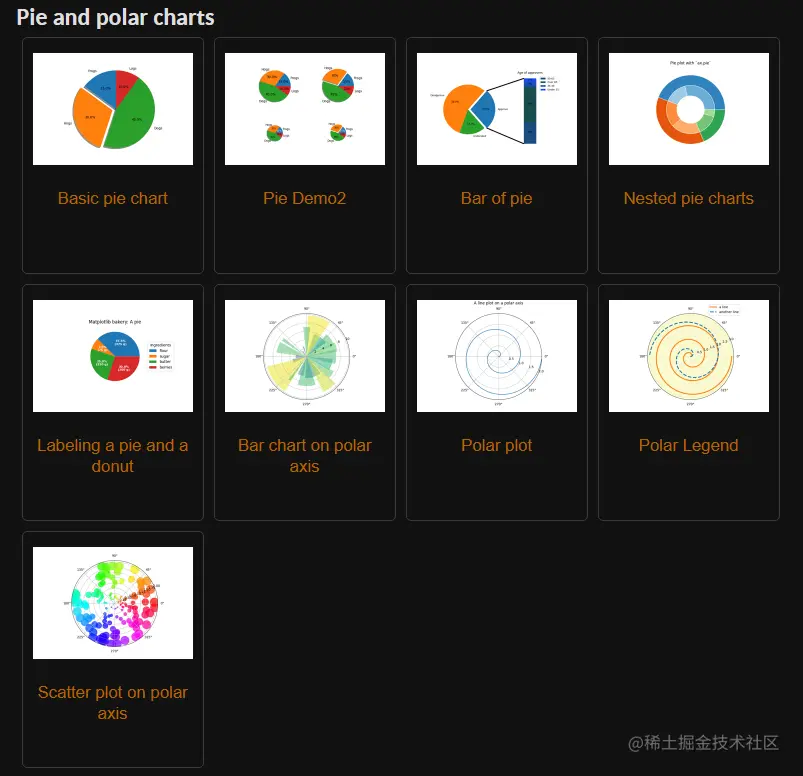In this issue , We will study in detail matplotlib Drawing pie chart related attributes of learning ,let's go~

# 1. Contour map Overview

• ## What is a pie chart ？

• The pie chart shows the ratio of the size of each item to the sum of the total items in a circle
• Pie charts are displayed through different sizes , To determine the proportion of each item
• Data markers of the same color in the pie chart form a data series
• Pie charts can be divided into three-dimensional pie charts 、 Composite pie chart 、 Split pie chart
• ## Pie chart common scenarios

• The pie chart can be used to determine the composition ratio of each part temporarily
• The pie chart can reflect the proportion of various indicators in a dimension in the overall
• Pie chart is suitable for only looking at the general proportion , Don't worry about data accuracy
• ## To draw an isopach

1. Import matplotlib.pyplot modular
3. call pyplot.pie() Draw the pie chart
4. call axis Method adjustment x/y The shaft spacing is equal
• ## The case shows

In this issue , We will apply pie chart to analyze the market share of operating system

• Case data preparation ： Use random.randint produce 5 A numerical

``````import numpy as np
size = np.random.randint(0,100,5)
Copy code ``````
• Draw the pie chart

``````import matplotlib.pyplot as plt\

plt.pie(size,labels=["Windows","MAC","Linux","Android","Other"])

plt.title(" Analysis on the proportion of mobile phone system ")

plt.show()
Copy code ``````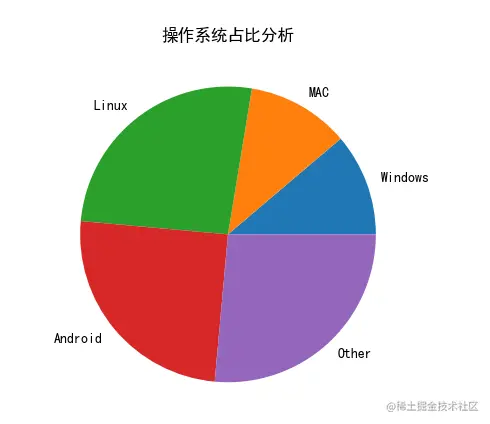# 2. Pie chart properties

• ## Set the color of the pie chart

• keyword ：colors
• Optional values ：None Or a list of colors
• The color list can consist of the following ：
• English words for color ： Like red "red"
• Abbreviations of words indicating color, such as ： Red "r", yellow "y"
• RGB Format ： Hexadecimal format, such as "#88c999";(r,g,b) Tuple form
• ## Set the label

• keyword ：labels
• The default is ：None
• You need to pass in a value in the form of a list
• ## Set the protrusion

• keyword ：explode
• The default is ：None
• Need to pass in list data
• If the value is set , The specified part is highlighted
• ## Set the fill percentage value

• keyword ：autopct
• The default is ：None
• Optional value form ：
• Format string, such as ：'%1.1f%%'
• function ： You can call the function content
• ## Pie chart rotation

• from x The shaft rotates counterclockwise ：startangle; The default is 0, Floating point type
• Specify the fractional direction clockwise ：counterclock; The default is True,bool type

• The default is False
• Draw a shadow under the pie chart
• Let's add some attributes in combination with the case in Section 1 , The proportion value needs to be displayed , Color displays the specified color , prominent MAC Proportion

``````plt.pie(size,labels=["Windows","MAC","Linux","Android","Other"],
autopct="%1.1f%%",
explode=[0,0.1,0,0,0],
colors=("r","blue","#88c999",(1,1,0),"0.5"))
Copy code ``````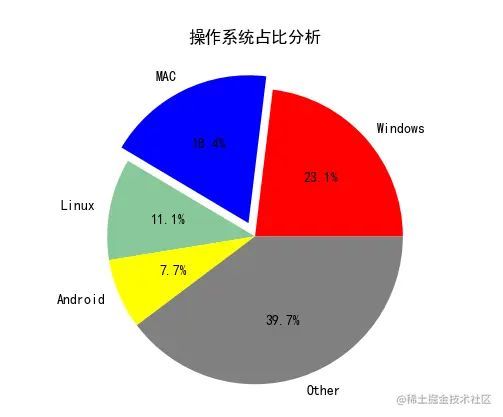# 3. Resize the pie chart

When we actually make pie charts , You will encounter changing the size of the pie chart , This is what we can do with the pie chart attribute keyword radius

besides , We also need to use textprops To control the size of the displayed labels

``````plt.pie(size,labels=["Windows","MAC","Linux","Android","Other"],autopct="%1.1f%%",
explode=[0,0.1,0,0,0],
Copy code ``````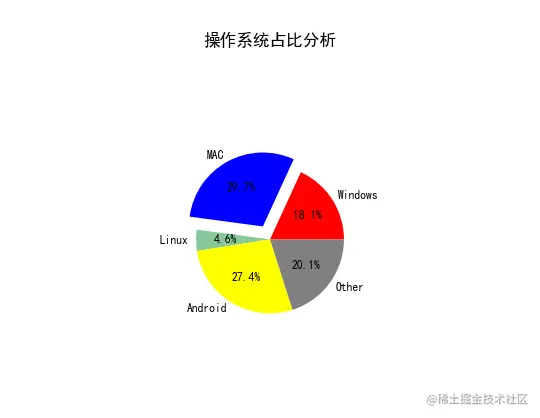When we show the proportion of each item in the pie chart , A set of legends will be added next to the chart .

• pyplot.pie() Method will return patchee.Wedge list 、 Text list and other data
• pyplot.legend() Methods the incoming wedge Element and specified labels label
• At the same time, you can work with legend() Method bbox_to_anchor To set the location of the legend
``````La = ["Windows","MAC","Linux","Android","Other"]

def f(pct,n):
num = int(round(pct*np.sum(n)))
return "{:.1f}%\n{:d}w".format(pct,num)

wedges ,text,autotexts =plt.pie(size,autopct=lambda pct: f(pct,size),
colors=("r","blue","#88c999",(1,1,0),"0.5"),textprops=dict(color='w'))

plt.legend(wedges,La,loc="right",bbox_to_anchor=(1,0,0.3,1))
Copy code ``````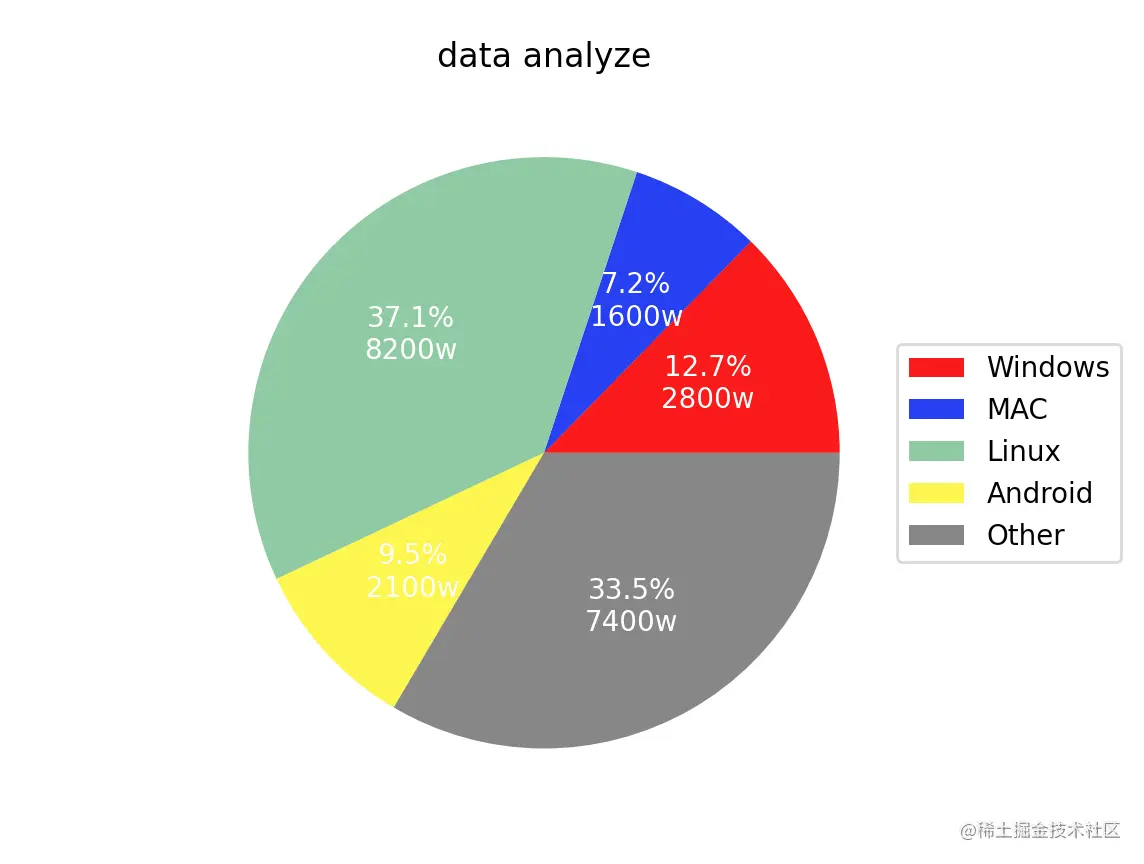# 5. Hollowed out pie chart

In the pie chart , We sometimes use nested hollowed out pie charts .

• Nesting can be called multiple times pyplot.pie() Method
• Hollowing out can be done with the help of pyplot.pie() attribute wedgeprops To set it up
• wedgeprops={"width":0.3,"edgecolor":'w'}
``````cmap = plt.get_cmap("tab20c")
plt.pie(size,
colors=("r","blue","#88c999",(1,1,0),"0.5"),textprops=dict(color='w'),wedgeprops=dict(width=0.3,edgecolor='w'))
plt.pie(size,
Copy code ``````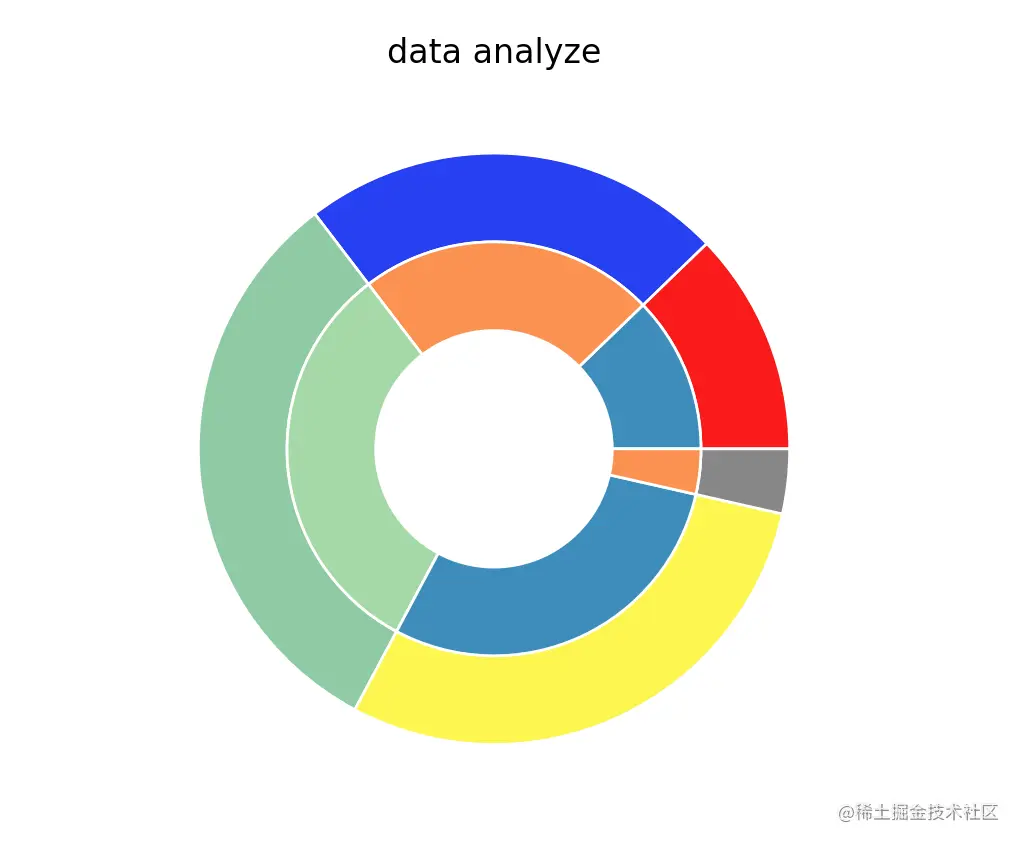# summary

In this issue , Yes matplotlib.pyplot Draw the pie chart pie() Learning related attributes . When drawing pie charts , We will change the size of the pie chart according to the actual needs , Nesting pie charts 、 Add graphics such as histogram to assist in viewing

The above is the content of this issue , Welcome big guys to praise and comment , See you next time ~